## Selina Concise Mathematics Class 10 ICSE Solutions Chapter 8 Remainder and Factor Theorems Ex 8B

These Solutions are part of Selina Concise Mathematics Class 10 ICSE Solutions. Here we have given Selina Concise Mathematics Class 10 ICSE Solutions Chapter 8 Remainder and Factor Theorems Ex 8B.

Other Exercises

Question 1.
Using the Factor Theorem, show that:
(i) (x – 2) is a factor of x3 – 2x2 – 9x +18. Hence, factorise the expression x3 – 2x2 – 9x + 18 completely.
(ii) (x + 5) is a factor of 2x3 + 5x2 – 28x – 15. Hence, factorise the expression 2x3 + 5x2 – 28x – 15 completely.
(iii) (3x + 2) is a factor of 3x3 + 2x2 – 3x – 2. Hence, factorise the expression 3x3 + 2x2 – 3x – 2. completely.
(iv) 2x + 7 is a factor of 2x3 + 5x2 – 11x – 14. Hence, factorise the given expression completely.
Solution: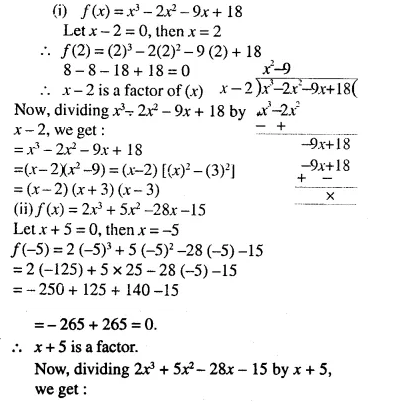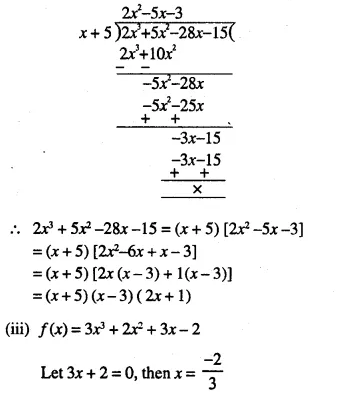Question 2.
Using the Remainder Theorem, factorise each of the following completely:
(i) 3x3 + 2x2 – 19x + 6
(ii) 2x3 + x2 – 13x + 6
(iii) 3x3 + 2x2 – 23x – 30
(iv) 4x3 + 7x2 – 36x – 63
(v) x3 + x2 – 4x – 4. (2004)
Solution: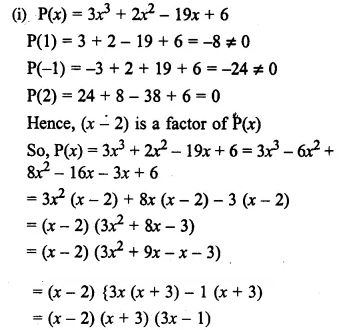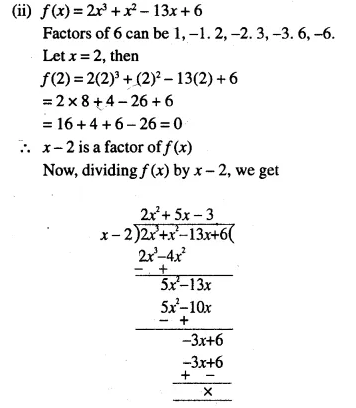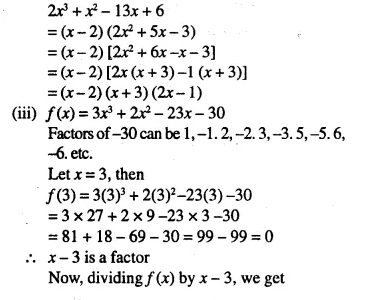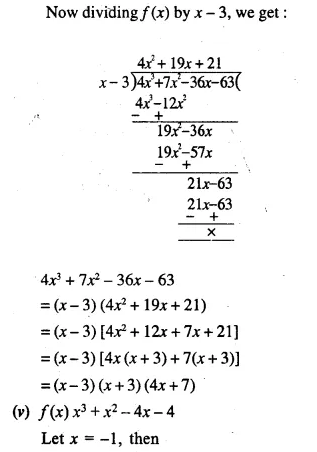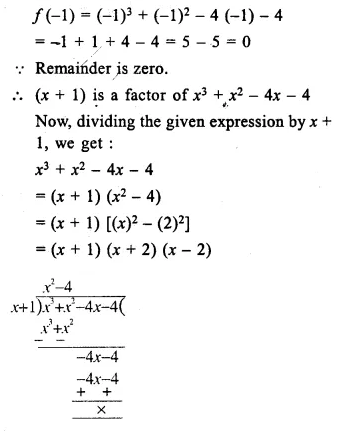Question 3.
Using the Remainder Theorem factorise the expression 3x3 + 10x2 + x – 6. Hence, solve the equation. 3x3 + 10x2 + x – 6 = 0
Solution: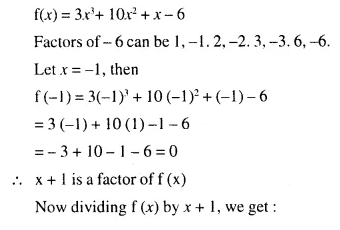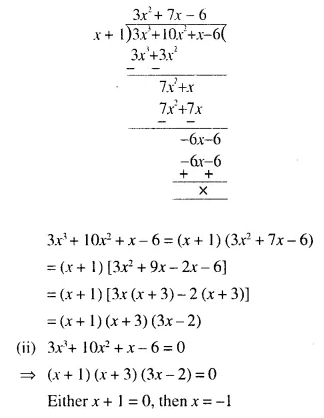Question 4.
Factorise the expression f(x) = 2x3 – 7x2 – 3x + 18. Hence, find all possible values of x for which f(x) = o.
Solution: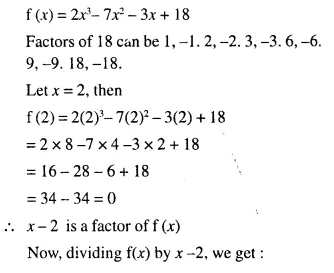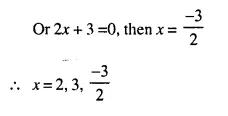Question 5.
Given that x – 2 and x +1 are factors of f(x) = x3 + 3x2 + ax + b; calculate the values of a and b. Hence, find all the factors of f(x).
Solution: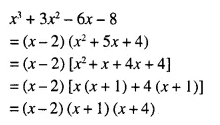Question 6.
The expression 4x3 – bx2 + x – c leaves remainders 0 and 30 when divided by x + 1 and 2x – 3 respectively. Calculate the values of b and c. Hence, factorise the expression completely.
Solution: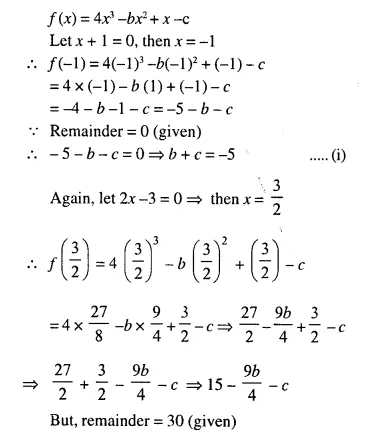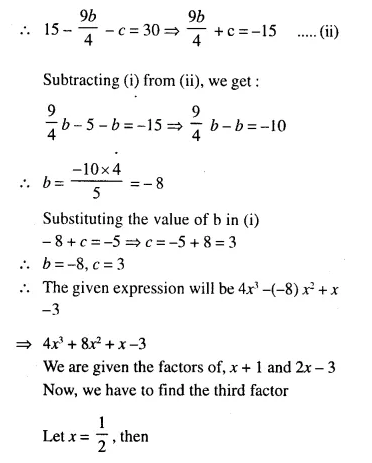Question 7.
If x + a is a common factor of expressions f(x) = x2 + px + q and g (x) = x2 + mx + n show that a = $$\frac { n – q }{ m – p }$$
Solution:Question 8.
The polynomials ax3 + 3x2 – 3 and 2x3 – 5x + a, when divided by x – 4, leave the same remainder in each case. Find the value of a.
Solution: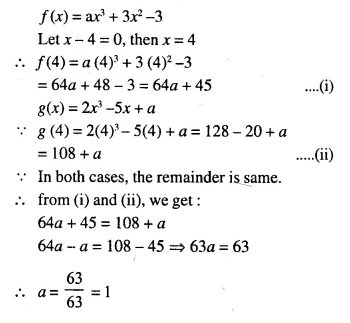Question 9.
Find the value of ‘a’ if (x – a) is a factor of x3 – ax2 + x + 2.
Solution:Question 10.
Find the number that must be subtracted from the polynomial 3y3 + y2 – 22y + 15, so that the resulting polynomial is completely divisible by y + 3.
Solution: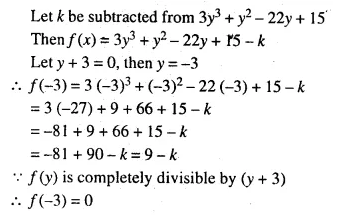⇒ 9 – k = 0 ⇒ k = 9
Hence 9 should be subtracted.

Hope given Selina Concise Mathematics Class 10 ICSE Solutions Chapter 8 Remainder and Factor Theorems Ex 8B are helpful to complete your math homework.

If you have any doubts, please comment below. Learn Insta try to provide online math tutoring for you.